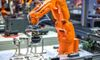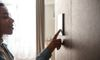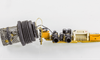# Forward Bias, Reverse Bias and their effects on Diodes08 Nov, 2021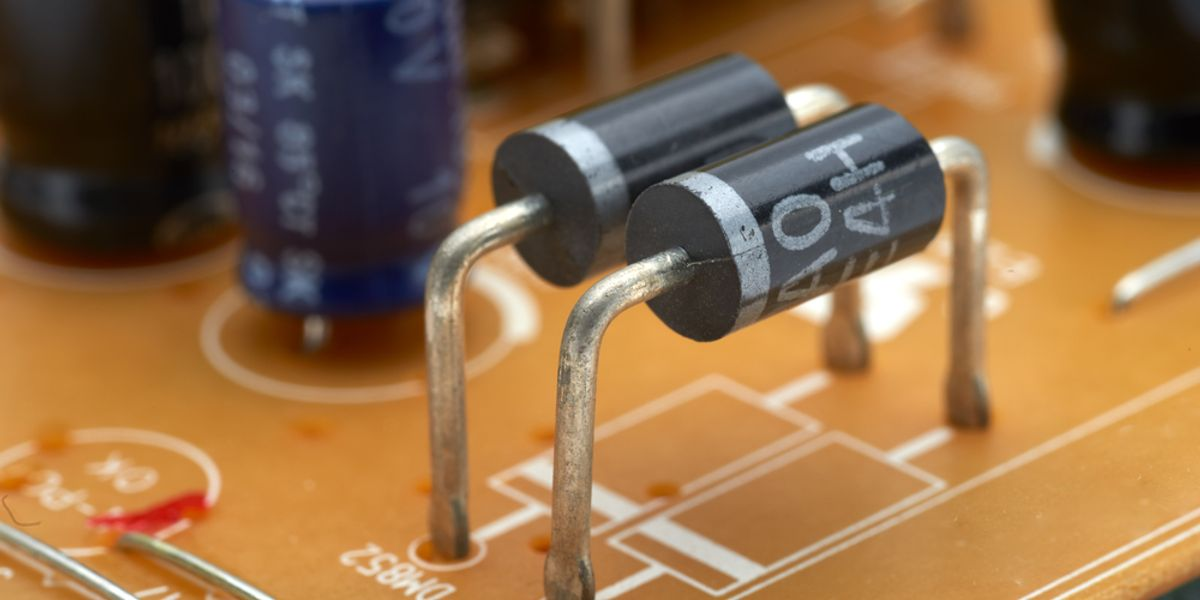## Diode is a simple electronic component that allows the flow of electric current in one direction while blocking it with high resistance in the other. This article explains the construction, working, biasing, and everything else you need to know about the most fundamental analog electronic component.

A diode is a two-terminal semiconductor component that allows the flow of electric current in a specific direction. Much like how check valves prevent the backflow of water, diodes are used in electrical circuits to prevent the backflow of current.

Diodes are denoted by a triangle having a line across one of its vertices, with each of its variations having a slightly different symbol. In this article, we shall explain the construction, working, biasing and some of the common application circuits of diodes. Several terms and definitions relevant to the topic have been covered.

## Construction of a p-n junction diode

When one side of a pure semiconductor material like Si or Ge is mixed with a Group 13 or Group 15 element, p-type (positive-type) or n-type (negative-type) semiconductor materials can be formed. Group 13 trivalent impurities like B, Al, Ga, or In form the p-region, whereas pentavalent elements like P, As or Sb, form the n-region. The process is called doping.

After doping, some free electrons from the electron-rich n-type semiconductor area undergo diffusion and bounce over to the hole-rich p-type semiconductor area and form a depletion region or a potential barrier.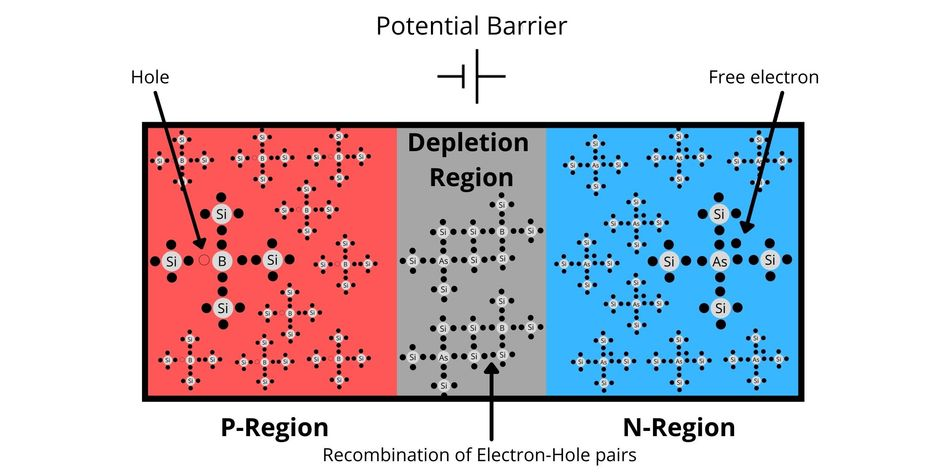Internal Construction of a p-n junction diode showing p-region. n-region and the depletion region

This region does not contain any mobile charges. Charged particles would require an additional force (voltage) to cross this barrier from now on due to a newly created obstruction. For a Silicon (Si) p-n junction, the typical value of this barrier is 0.7 V, and for p-n junctions developed with Germanium (Ge), it's 0.3 V. This voltage is also known as the cut-in voltage.

Before we proceed, we need to define another term that will be the centre of discussion for the next few sections.

Biased Diodes: Application of a fixed DC voltage or current between or through two different but fixed points in an electronic circuit is known as bias or biasing. It is usually done for testing the devices or creating optimal working conditions for the circuit.

The i-v characteristics of a diode closely follow this equation during operation in forward bias: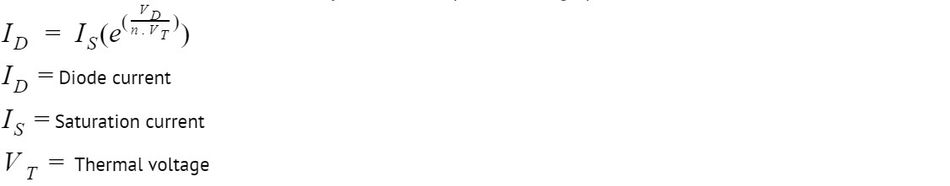The same equation can be the basis for plotting the i-v characteristics of a practical diode. Note: the scale has been compressed and expanded appropriately for the purpose of explanation.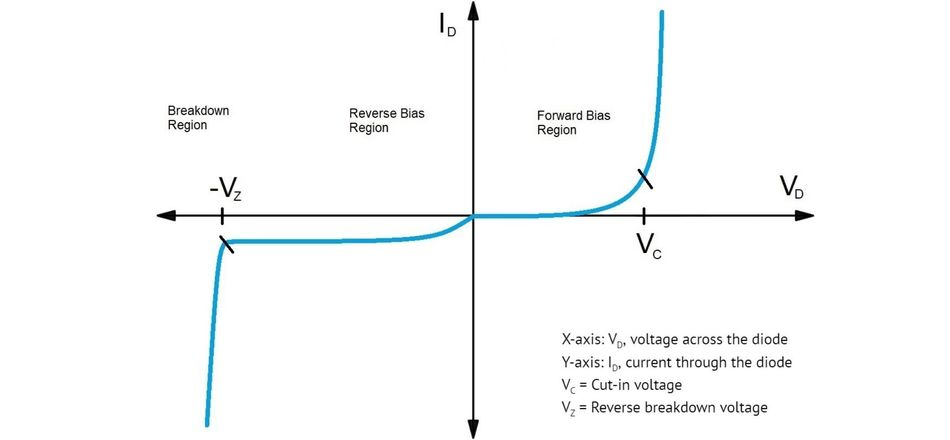I-V characteristics of a practical diode

The graph of a practical diode is a deviation from the practical diode characteristics, which require it to switch on and conduct infinite current or switch off and block the current completely without any voltage drop.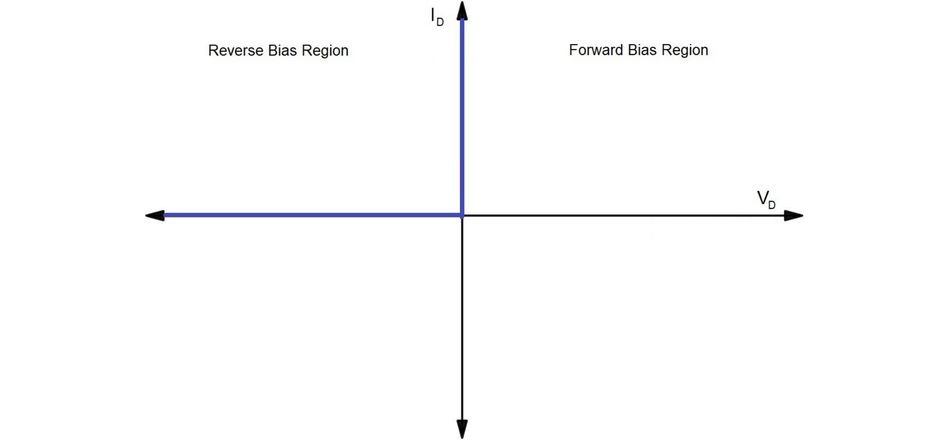I-V characteristics of an ideal diode

The diodes that exhibit such characteristics are used in simulation and mathematical analysis. They are called ideal diodes and are purely hypothetical.

## What is Forward Bias?

When the potential applied at the anode (p-side) of a practical diode is higher than the potential applied at the cathode (n-side) by at least the cut-in voltage, the diode is said to be in a forward bias.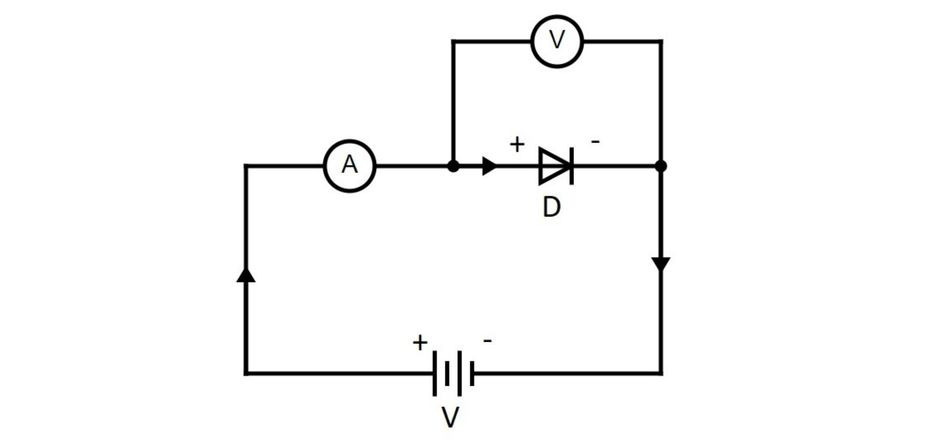Forward Bias circuit for diode D

It can be clearly seen from the diagram that the positive end of the battery is connected to the positive terminal of the diode, and the negative end of the battery is connected to the negative terminal of the diode.

### Properties of p-n Junction in Forward Bias

The externally applied voltage in forward bias sets up an electric field in the opposite direction to that of the potential barrier. As the value of external voltage increases from 0 to cut-in voltage, the width of the potential barrier decreases. The value of current (leakage current) flowing through the diode is negligibly small in this case and is only due to some electrons that manage to jump across the potential barrier.

When the external voltage is equal to the cut-in voltage, the potential barrier vanishes, thereby breaking the barrier stopping the free movement of current. As the voltage increases even more, the current rises exponentially until the diode enters into a fully conducting zone.

At this point, a practical diode can be modelled as a series combination of a voltage source in series with a resistor. The value of the voltage is extremely small and is equal to the cut-in voltage. Resistance, too, is small in magnitude.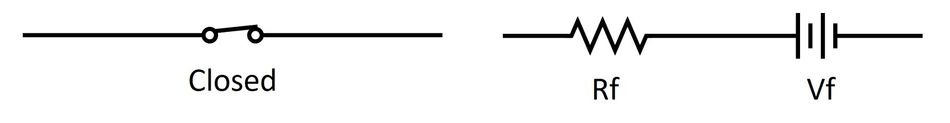An ideal diode in forward bias behaves like a closed switch (left), and a practical diode is equivalent to a small voltage source in series with small resistance

VD = VF + IDRF is the voltage that appears across the diode and depends on the diode current. Since the value of resistance is low, the IDRF  term will not change a lot.

Coming to the forward voltage drop VF, let I1 and I2 be the diode currents corresponding to the applied diode voltages V1 and V2 across two diodes.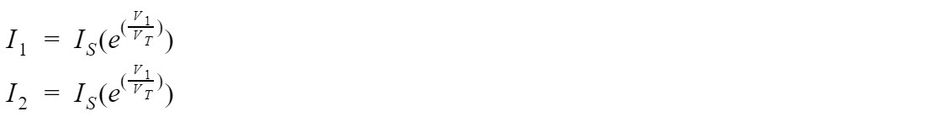The ratio of the equations give,Which can be rewritten as: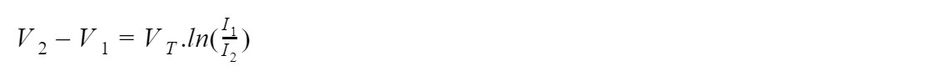The voltage/current difference equation suggests that even for a drastic change in the value of current, the voltage drop across the diode will not change much, which is a simple consequence of a logarithmic relationship.

Hence, during the analysis of a diode in forward bias, the on-state voltage drop can be considered almost constant for a reasonably significant change in diode current.

## What is Reverse Bias?

When the potential applied at the cathode (n-side) is higher than the potential applied at the anode (p-side), the diode is said to be in a reverse bias.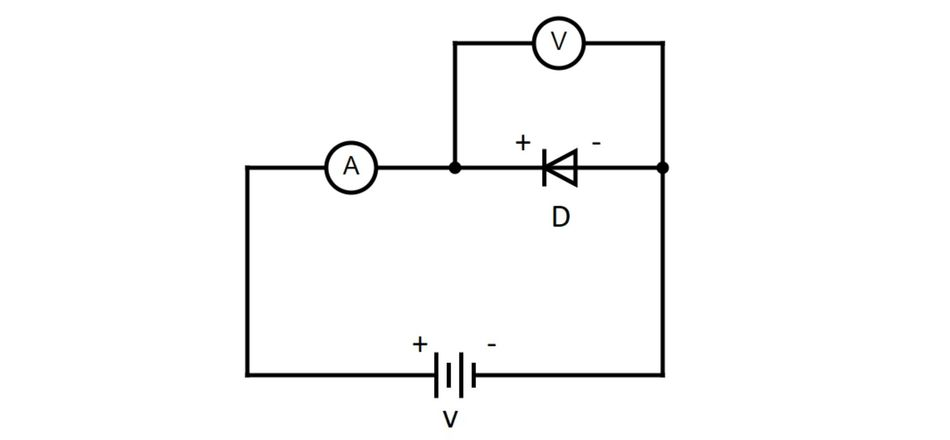Reverse Bias Circuit for Diode D

The positive end of the battery is connected to the negative terminal of the p-n junction diode, and the negative end of the battery is connected to the negative terminal of the p-n junction diode.

### Properties of p-n Junction in Reverse Bias

As the reverse voltage is increased from zero, a small reverse current starts flowing through the semiconductor device. This electric current is due to the minority charge carriers present in the diode. The value of this reverse current is sufficiently small (compared to the forward current) for us to completely ignore its effect in practical applications.An ideal diode in forward bias behaves like a closed switch (left), and a practical diode is equivalent to a small voltage source in series with small resistance

Even after increasing the value of voltage by a considerable amount, the value of reverse current isn't affected much and remains almost constant. This is the reason why it is also known as the saturation current. Having said that, the value of saturation current can change drastically with temperature.

### Reverse Breakdown region

If we keep increasing the value of reverse voltage, the size of the depletion layer will keep growing. This increase in the number of immobile ions causes the electric field in the depletion region to get stronger. The minority charge carriers in the vicinity will get accelerated and can collide with Si atoms, thereby knocking off a valence electron. Once this happens, there will be two free mobile electrons. These electrons further collide with other Si atoms and knock more valence electrons from other atoms kicking off what we can call a chain reaction.

In the absence of a current limiting resistor, the value of current and power dissipation can exceed the safe limits and permanently damage the semiconductor diode. This phenomenon is known as an avalanche breakdown, and the threshold reverse voltage at which this occurs is known as the breakdown voltage.

For a normal diode, operation in such conditions should be avoided. But there are some diodes that are specifically developed for operating in the breakdown region. Such diodes are called Zener diodes or breakdown diodes. We will have a look at the working of the Zener diode in a later section.

## Forward Bias vs Reverse Bias

Here is a table that summarises the comparison between diodes in Forward Bias vs Reverse Bias.

 Parameter Forward Biased p-n junction Reverse Biased p-n junction Polarity of external voltage Higher voltage at the anode and a lower voltage at the cathode Lower voltage at the anode and a higher voltage at the cathode Effect on potential barrier Depletes Strengthens Resistance offered Low (like a conductor) Very High (like an insulator) Current through the diode High Very Low Voltage across the diode Low High Diodes operating in such conditions LEDs, diodes in rectifiers Zener diodes, Photodiodes

## Applications of silicon diodes

Semiconductor diodes find their application in a variety of different circuits that are a part of appliances we use in our day to day life. Diodes can be combined with other electrical/electronic components to develop circuits that can control and convert electrical signals.

### Rectifiers

The rectifier is a circuit used to convert AC power into DC directly. It is one of the most important applications of a diode and is the basic building block of power supplies. Rectifiers can be found in chargers, Switch Mode Power Supplies (SMPS), and a lot of other places where a conversion from AC to DC power takes place.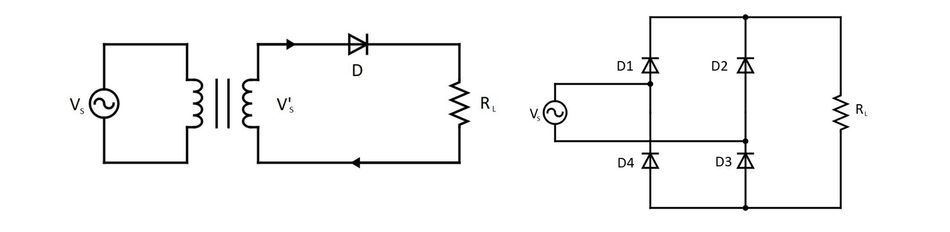Circuit diagram of a Single Phase Half Bridge Rectifier using a single diode (left) and Single Phase Full Bridge Rectifier using four diodes (right)

The working of the Single Phase Half Bridge Rectifier in the figure above is pretty straightforward. During the positive half cycle, the diode D is forward biased and conducts. During the negative half-cycle, it is reverse biased and blocks the current. Simpler versions of the rectifiers made using a single diode offer very low efficiency and introduce severe harmonics.

In the Single Phase Full Bridge Rectifier circuit, during the positive cycle of the AC voltage source, the current flows through diode D1 into the load Rl and returns through D3. When the source voltage is reversed, the current flows through D2 into Rl and then returns through D4.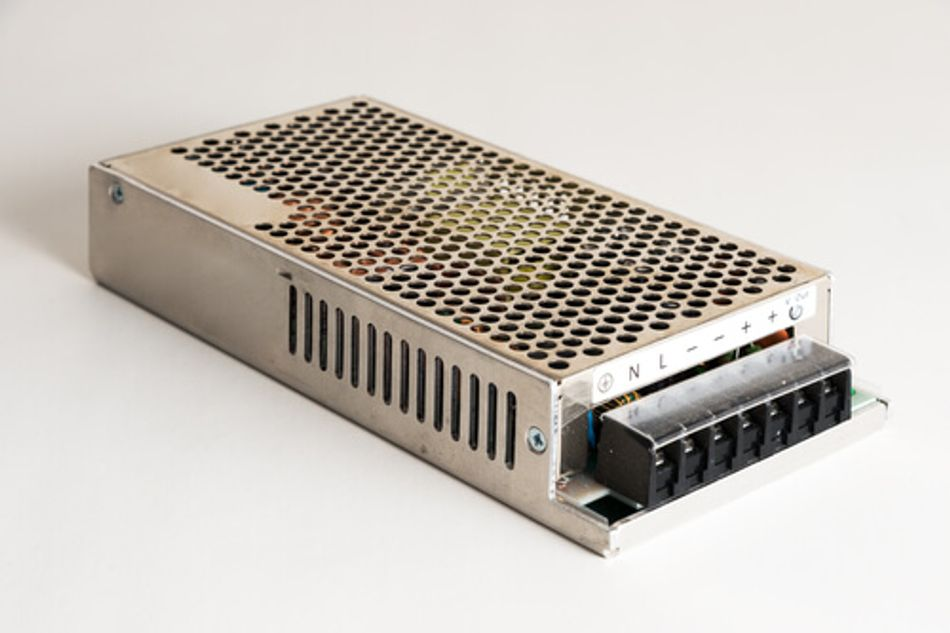SMPS converts AC power into regulated DC power

A common misconception about diodes or electronics, in general, is that they can not handle high electrical power. But by implementing some constructional and compositional changes, power diodes can be developed, which can easily withstand a voltage in the order of several kilo-volts and current in the range of several kilo-amperes.

The growing interest in the application of electronics to control and convert electrical energy has given birth to an entirely different branch of electrical engineering known as power electronics.

### Voltage regulators

Zener diodes are used as voltage regulators in circuits that are intolerant to voltage fluctuations. The component that needs to be protected from voltage changes is connected in parallel with a Zener diode. In the circuit shown below, the voltage across the Zener diode is adjusted such that it always operates in the reverse breakdown region.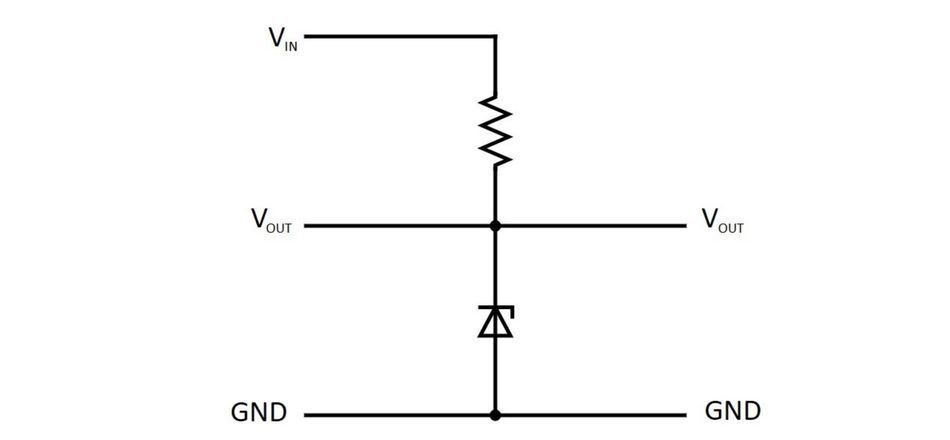Circuit diagram of Zener voltage regulator

Whenever there is a spike in voltage, the Zener diode bypasses the excess current to maintain the voltage within the safe limits. Zener diodes have largely been replaced by Integrated Circuits (ICs) that offer much higher flexibility lately.

### Logic Gates

Everything from calculators to computers is built with digital ICs, and digital ICs are built with digital logic. Logic gates are the basic building blocks of any digital electronic system. Before the introduction of modern logic families like Complementary Metal Oxide Semiconductor (CMOS) technology, diodes were used to implement the logic gates. Here are the circuit diagrams showcasing the implementation of AND and OR logic using diodes: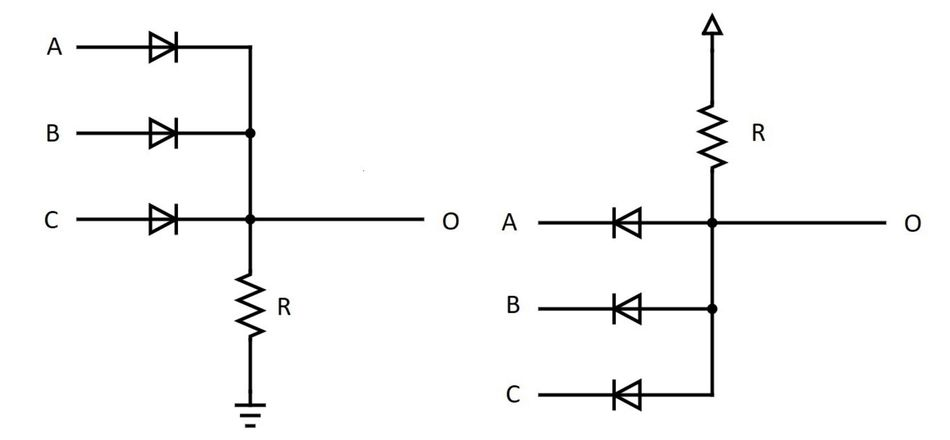Realising AND (left) and OR (right) logic gates using diodes and resistors

### Waveshaping circuits

There are numerous Clipper and Clamper circuits that make use of diodes and other basic electrical elements like resistors, capacitors and inductors to shape the signals. Here is a circuit diagram of a positive clamper. A clamper is a circuit that provides an offset to the input voltage signal.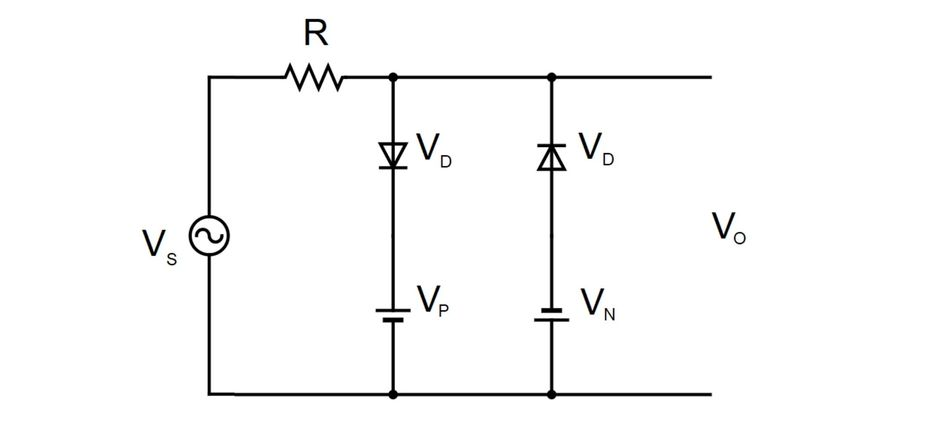Positive Clamper circuit

As the AC Voltage source Vs is switched on, the capacitor connected to it starts charging. Once the circuit reaches the steady-state, the capacitor is charged to Vc volts. Since no path is available for the capacitor to discharge, Vc gets added to the source voltage in the subsequent cycles, and the resulting voltage appears at the output.

Clipper, on the other hand, is a circuit used to limit the value of input voltage between a certain range at the output. Here is a circuit diagram of a positive-negative clipper circuit.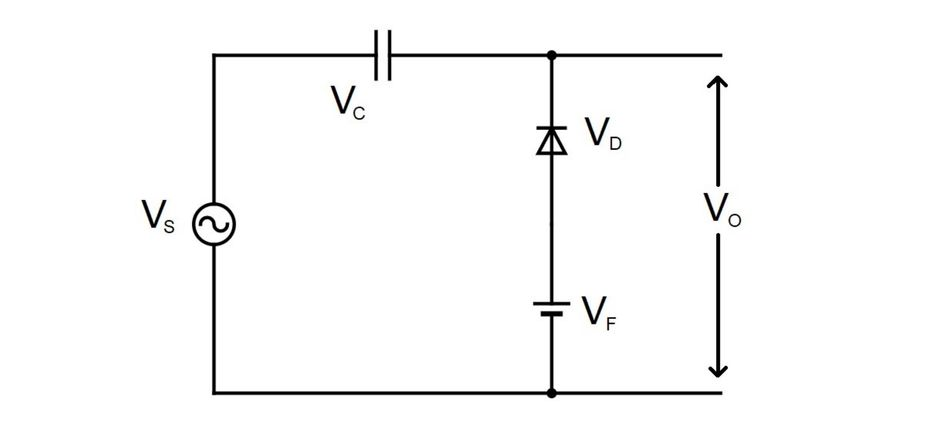Positive-Negative Clipper circuit

Until the value of source voltage Vs approaches Vp, the diode is reverse biased. As soon as Vs exceeds Vp, the diode is forward biased and blocks any voltage higher than Vp from appearing across the output. As the polarity of Vs reverses, the same effect is enacted by the other diode. Thus, making Vo range-bound between Vp and Vn.

These circuits are collectively called waveshaping circuits and are used in filters, integrators, differentiators, wave modifiers, multivibrators or anywhere signals need to be changed.

## Special Diodes

Apart from the prominent properties that diodes exhibit under forward bias/reverse bias, they can also be designed to fulfil some other special purposes. These diodes are still made by a combination of p-type material and n-type material but can behave very differently.

Light Emitting Diodes (LEDs): LEDs as an electronic component need no introduction. These devices are present everywhere and are among the most efficient illuminating solutions known to us.

Just like the regular diode, when LEDs are forward biased, both majority carriers and minority carriers participate in the conduction of current. In regular diodes, this process is accompanied by the generation of heat, but in LEDs, light is also produced.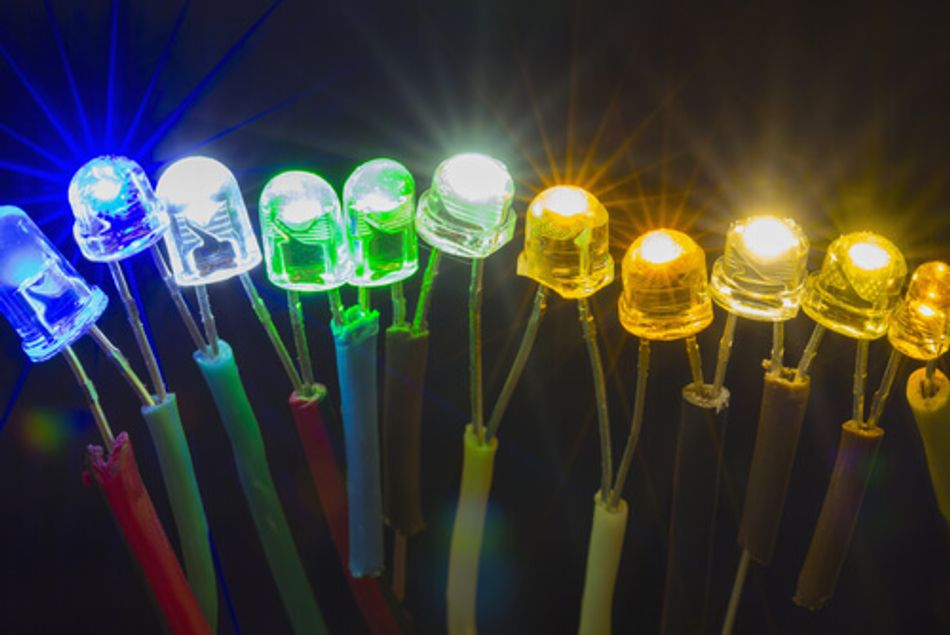LEDs come in different shapes, sizes and colours

This phenomenon can also be explained with the help of an energy band diagram. Electrons are present in the conduction bands and holes in the valence band in the crystal structure. When the recombination happens, the negative charges release the extra energy and settle to the valence band. Depending on the bandgap width, the colour of the emitted electromagnetic wave changes. Gallium Arsenide (GaAs) and Gallium Phosphide (GaP) are the semiconductors used in LEDs in place of Si/Ge.

Photodiodes: In simple terms, photodiodes are electronic devices that convert light into electricity. Their working principle is exactly the opposite to that of LEDs. When photons are incident on the junction, the electrons present in the valence band of the crystalline lattice are excited and jump to the conduction band and participate in the flow of electric current. Only if we use the photodiode in the reverse bias condition, the depletion layer will be large and allow more recombination to occur.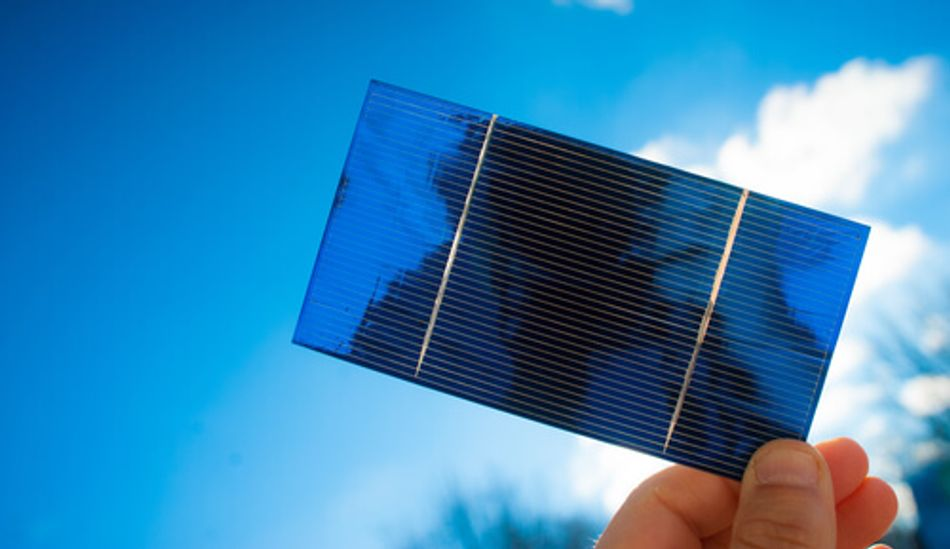A photovoltaic cell made with an array of photodiodes

These devices can be used in automatic street lights to sense ambient light levels. Specially designed photodiodes for converting sunlight into electricity are known as solar cells and are leading the green energy revolution.

Schottky Diodes: A Schottky Diode or Schottky Barrier Diode is a special diode that can rapidly switch between conduction and blocking state depending on the forward bias voltage or reverse bias voltage applied. These diodes also have a relatively lower on-state voltage drop.

During the manufacturing of this diode, one side of the junction is formed by metal, and the other is formed by an n-type semiconductor. The n-type region is moderately doped. The conduction of current in Schottky diodes is due to majority carriers only, and hence minority carrier charge storage effect is not observed, which ultimately improves the dynamic response.

Varactors: Varactors get their name from their ability to offer a variable capacitance (reactance) based on the strength of the applied reverse bias. As the magnitude of the reverse voltage is changed, the width of the depletion layer changes, which results in a change in capacitance. Voltage-controlled oscillators, tuners, parametric amplification circuits etc., make extensive use of varactors.

## Conclusion

Different diodes are used in power supplies, switches, protection circuits, solar cells, isolators, sensing applications, logic design, lighting, and more. If you are using any electronic equipment, chances are it contains at least one p-n junction diode. It is interesting to note how a simple electronic component that allows a unidirectional current flow can find such a wide range of domestic and industrial applications.

## References

 Sedra, A. S., Smith, K. C., Carusone, T. C., & Gaudet, V. (2021). Microelectronic circuits. Oxford University Press.

 Kaiser, C. J. (1999). The diode handbook. CJ Pub.

 Diodes Explained - The basics how diodes work working principle pn junction. (2020). Retrieved from https://www.youtube.com/watch?v=Fwj_d3uO5g8&t

## More by Ravi Rao

Meet Ravi, a backend engineer at the world's largest IT services company. He collaborates with solution architects and clients on a massive digital transformation project. Graduating in electrical engineering from a public university in India, Ravi ranked among the top 0.32% of 18,000+ students. His...